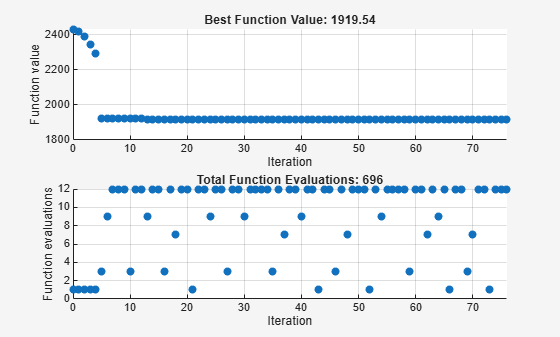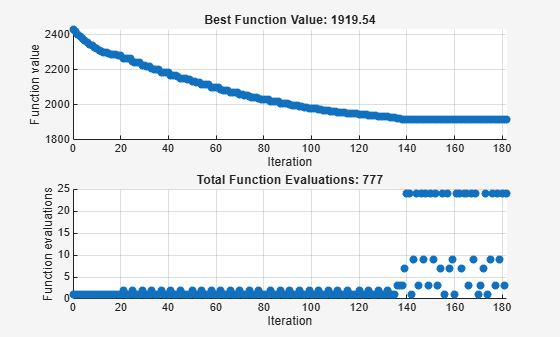# Search and Poll

In addition to polling the mesh points, the pattern search algorithm can perform an optional step at every iteration, called search. At each iteration, the search step applies another optimization method to the current point. If this search does not improve the current point, the poll step is performed.

### Search Using a Poll Method

The following example illustrates the use of a search method on the problem described in Constrained Minimization Using patternsearch and Optimize Live Editor Task. In this case, the search method is the MADS Positive Basis 2N poll. For comparison, first run the problem without a search method.

```x0 = [2 1 0 9 1 0]; Aineq = [-8 7 3 -4 9 0]; bineq = 7; Aeq = [7 1 8 3 3 3; 5 0 -5 1 -5 8; -2 -6 7 1 1 9; 1 -1 2 -2 3 -3]; beq = [84 62 65 1]; options = optimoptions('patternsearch',... 'PlotFcn',{@psplotbestf,@psplotfuncount}); [x,fval,exitflag,output] = patternsearch(@lincontest7,x0,... Aineq,bineq,Aeq,beq,[],[],[],options);```
```Optimization terminated: mesh size less than options.MeshTolerance. ```To use the MADS Positive Basis 2N poll as a search method, change the `SearchFcn` option.

```rng default % For reproducibility options.SearchFcn = @MADSPositiveBasis2N; [x2,fval2,exitflag2,output2] = patternsearch(@lincontest7,x0,... Aineq,bineq,Aeq,beq,[],[],[],options);```
```Optimization terminated: mesh size less than options.MeshTolerance. ```Both optimizations reached the same objective function value. Using the search method reduces the number of function evaluations and the number of iterations.

```table([output.funccount;output2.funccount],[output.iterations;output2.iterations],... 'VariableNames',["Function Evaluations" "Iterations"],... 'RowNames',["Without Search" "With Search"])```
```ans=2×2 table Function Evaluations Iterations ____________________ __________ Without Search 1462 136 With Search 1283 118 ```

### Search Using a Different Solver

`patternsearch` takes a long time to minimize Rosenbrock's function. The function is

`$f\left(x\right)=100{\left({x}_{2}-{x}_{1}^{2}\right)}^{2}+\left(1-{x}_{1}{\right)}^{2}.$`

Rosenbrock's function is described and plotted in Solve a Constrained Nonlinear Problem, Solver-Based. The minimum of Rosenbrock's function is 0, attained at the point `[1,1]`. Because `patternsearch` is not efficient at minimizing this function, use a different search method to help.

Create the objective function.

`dejong2fcn = @(x)100*(x(2)-x(1)^2)^2 + (1-x(1))^2;`

The default maximum number of iterations for patternsearch with two variables is 200, and the default maximum number of function evaluations is 4000. Increase these values to `MaxFunctionEvaluations` = 5000 and `MaxIterations` = 2000.

`opts = optimoptions('patternsearch','MaxFunctionEvaluations',5000,'MaxIterations',2000);`

Run patternsearch starting from `[-1.9 2]`.

```[x,feval,eflag,output] = patternsearch(dejong2fcn,... [-1.9,2],[],[],[],[],[],[],[],opts);```
```Maximum number of function evaluations exceeded: increase options.MaxFunctionEvaluations. ```
`disp(feval)`
``` 0.8560 ```

The optimization did not complete, and the result is not very close to the optimal value of 0.

Set the options to use `fminsearch` as the search method, using the default number of function evaluations and iterations.

`opts = optimoptions('patternsearch',opts,'SearchFcn',@searchneldermead);`

Rerun the optimization.

```[x2,feval2,eflag2,output2] = patternsearch(dejong2fcn,... [-1.9,2],[],[],[],[],[],[],[],opts);```
```Optimization terminated: mesh size less than options.MeshTolerance. ```
`disp(feval2)`
``` 4.0686e-10 ```

The results are much better when using this search method. `fminsearch` is more efficient at getting close to the minimum of Rosenbrock's function.

## SupportGet trial now